# FKG inequality

(diff) ← Older revision | Latest revision (diff) | Newer revision → (diff)

Fortuin–Kasteleyn–Ginibre inequality

An inequality [a3] that began a series of correlation inequalities for finite partially ordered sets. Letbe a finite partially ordered set ordered by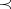(irreflexive, transitive) witha distributive lattice:,, and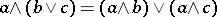for all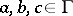. Suppose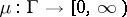is log supermodular:and thatandare non-decreasing:The FKG inequality is: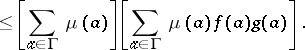Ifis a Boolean algebra andis a probability measure on, the inequality is, where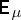denotes mathematical expectation.

Related inequalities are discussed in [a1], [a2], [a4], [a5], [a6], [a7], [a8], [a9].

How to Cite This Entry:
FKG inequality. P.C. Fishburn (originator), Encyclopedia of Mathematics. URL: http://encyclopediaofmath.org/index.php?title=FKG_inequality&oldid=14368
This text originally appeared in Encyclopedia of Mathematics - ISBN 1402006098